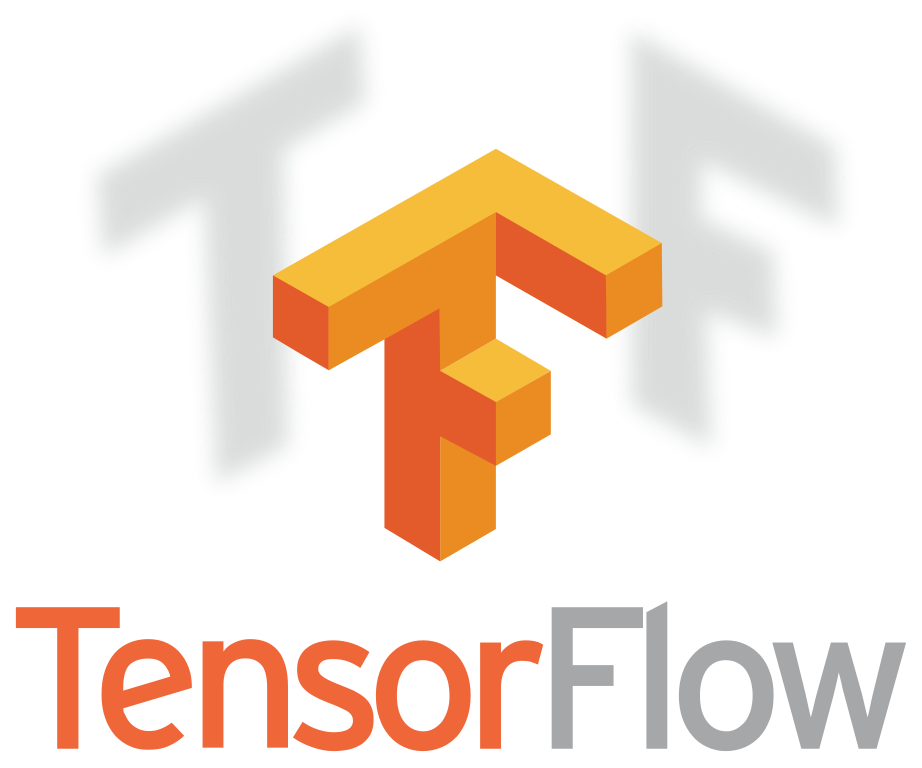시간 제한 메모리 제한 제출 정답 맞은 사람 정답 비율
1 초 512 MB 11 11 11 100.000%

## 문제Everybody knows that you are a TensorFlow fan. Therefore, you’ve been challenged to recreate the TensorFlow logo from two projections.

Consider that you have a 3D volume, n×m×h, and two projections (two matrices with dimensions n×m and n × h with elements 0 and 1). You are asked to compute a possible sets of cubes that must be placed inside the 3D volume such that the 3D object created with the cubes throws the shadows specified by the projection-matrices, when the light comes from left and front. If it is not possible, just print −1. If it is possible you must find exactly two sets, one with the maximum amount of cubes and one with the minimum amount. You can assume there is no gravitation (the cubes are located inside the 3D volume exactly where they are placed, without requiring any support). We assume that 1 represents shadow and 0 represents light.

If there are multiple such solutions, you must output the minimum lexicographic one. One solution A is lexicographically smaller than another solution b if the first number that differs between the two solutions is smaller in a than in b.

For example, solution [(0, 0, 0),(1, 1, 1)] is smaller than [(1, 1, 1),(0, 0, 0)].

## 입력

The first line contains three integers separated by a single space n, m, h (1 ≤ n, m, h ≤ 100) — the volume dimensions.

Each of the next n lines contains m characters, each being either 1 or 0 representing either a shadow area (1) or a light area (0), describing the projection from the light in the front.

Each of the next n lines contains h characters, with the same format as above, describing the projection from the light on the left.

## 출력

The output should contain on the first line one number, either −1 if there is no solution or kmax representing the maximum number of cubes we can assign in the volume that will generate the two projections given in the input.

The next kmax lines should contain triplets of numbers x, y, z (0 ≤ x < n, 0 ≤ y < m, 0 ≤ z < h) representing the cubes chosen in the lexicographically smallest solution with maximum number of cubes.

Then, only if there is a solution, one more line follows containing kmin, the minimum number of cubes we can assign in the volume that will generate the two projections given in the input.

After that, the next kmin lines should contain triplets of numbers x, y, z (0 ≤ x < n, 0 ≤ y < m, 0 ≤ z < h) representing the cubes in the lexicographically smallest solution with minimum number of cubes.

## 예제 입력 1

5 3 3
111
010
010
010
010
111
100
110
100
100


## 예제 출력 1

14
0 0 0
0 0 1
0 0 2
0 1 0
0 1 1
0 1 2
0 2 0
0 2 1
0 2 2
1 1 0
2 1 0
2 1 1
3 1 0
4 1 0
8
0 0 0
0 1 1
0 2 2
1 1 0
2 1 0
2 1 1
3 1 0
4 1 0


## 예제 입력 2

2 2 2
00
00
11
11


## 예제 출력 2

-1


## 예제 입력 3

2 3 2
101
011
10
11


## 예제 출력 3

6
0 0 0
0 2 0
1 1 0
1 1 1
1 2 0
1 2 1
4
0 0 0
0 2 0
1 1 0
1 2 1


## 노트

A cube at coordinates (x, y, z) will generate a shadow at line x and column y in the n × m projection and line x and column z in the n × h projection (indexed from 0).